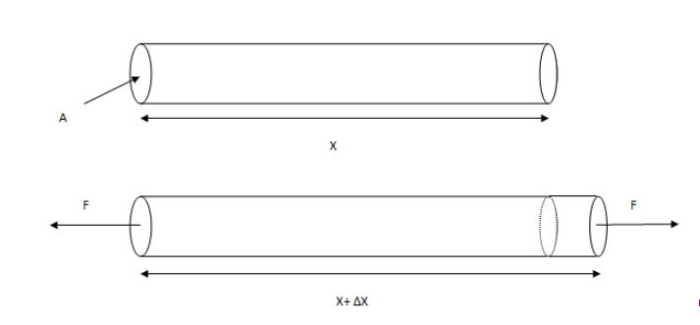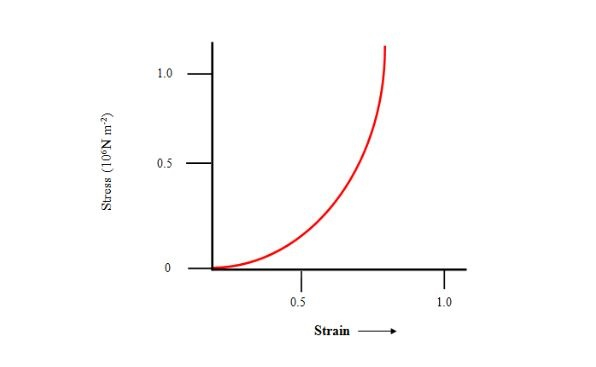# Stress and Strain

## Introduction

The application of stress is used to deform an object with the applied pressure which is external. According to physics, the ratio of stress to strain will remain consistent. Elasticity is one of the considerably significant mechanical effects to evaluate when selecting the right object for its application. Additionally, in this article, the relationship between stress and strain has been highlighted by making appropriate comprehension of Hooke’s laws.

## Information Regarding Stress

The application of force that is used to deform an object by applying it from the external force is known as stress. The amount of applied force that is used to make deformation of an object where the shape and size are accurate, stress is ought to be applied. Additionally, “Stress = force / cross-sectional area”.

## Information Regarding Strain

Based on the comprehensive knowledge delivered by physics, the deformation experienced by an object with the applied direction of force is the strain. Generally, the initial proportions of the object divide Strain. “Strain is described as an extension to the size in a separate unit.” Strain is the ratio of the quantity of deformation participated by the body in the directive of applied strength to the initial altitudes of the object.## Stress-Strain Curve

The curve of stress-strain is building a relationship for understanding how the applied force in the area per unit works with the amount of applied force to the magnitude. An object’s stress-strain curve illustrates the connection between stress and strain with the applied force to deform an object. The values of strain are extemporised on the curve corresponding to the pressure incurred by additional limitations on the entity.

The relationship between “strain and stress” relies on an object that delivers the properties of stress underneath tensile stress which is an experimental entity. In an ordinary test of the belongings of tensile, a complicated force stretches a wire to comprehend the strain (Tu et al. 2020).

This process is common as strain can only be measured with the help of the amount of applied force on an object for the deformation property. The involved strength is slowly inflated in stages and the distinction in measurement can be described through the “curved line stress and strain”.The curved line is a diagram which is a property of the stress-strain relationship. This diagram is utilised to understand the amount of stress that is applied is also distinctive. One basic component in the tutorial on the technicians of deformable objects is the resistive belongings of the items. These belongings relate the pressures to the strains and can exclusively be decided by investigation.

Additionally, an object can face two distinctive types of strain that is also depending on the application of stress. The most common method of stress and strain is a subject to test the tensile force applied to an object.

On the same measurement and cross-section, the proportional relation can make the approach of the object to face the deformational forces. The strain supplements at a more instantaneous rate than priority which embodies itself as a soft “flattening of the curve in the stress and strain” diagram.

## Hooke’s Law

The principles of Hooke’s law are about to application of force that is required to extend or compress the elasticity with the amount of distance that is certainly symmetrical to the travelled distance. The extended amount of force is reliable on the compression power of the elastic object with the symmetrical relation with the distance. Moreover, the proportional relation can make the objects durable during the applied stretches .

Additionally, Hooke’s law states that for most items, greater differences in height will complete greater inner strengths. Stress in this case is directly symmetrical to the applied quantity of deformed stress which is also known as stress.

## Proportional Limit

All the items conduct like an elastic thing over a specific field due to the elasticity it has. This field contrasts with different metals and is simulated by elements such as automatic effects, atmospheric disclosure (corrosion) heat therapy, and operating temperature (Wang et al. 2020). These are the factors, which is relying upon the facilities of an item while the stretches are applied with the force.

## Elastic Point

In the practice, the applied amount of the tensile force that is increasing with the proportional limit. The amount of applied force is equal to the faster rate of the stress that the creating the manifest where the mild curve in the stress and the applied strain. The change in the dimension is making the elasticity limit converted reversible and temporary (He, Liang & Huang, 2018). How considerably force on the object background can be calculated employing units of stress.

## Conclusion

In this tutorial, the relation between the strain and stress is highlighted with the application of the conductions of the elasticity. Based on the object different forces are applied from the external side to deform a material. Further, stress can be classified into three varieties counting on the demand of the deforming intimidation acting on the object. The relation between the two is used applied in physics in order to comprehend the deformation force that can occur continues with stress that has a rate of increase in amount.

## FAQs

Q.1. What is Elastic Limit?

Ans. The elastic limit is the stress that is applied with the maximum force that can endure the substance of the permanent deformation. The applied pressure on an object can be removed fully with the changing of its original position.

Q.2. What causes stress?

Ans. The aim of applying deformation force to change the location is ultimate within the application of external force. This external force of deforming an item is known as stress.

Q.3. What is the relation between Stress and Strain?

Ans. Both properties, stress and strain are symmetrical to each other directly for the convection of the boundary of elasticity. A curve line is constructed to understand the relation between “stress and strain”.